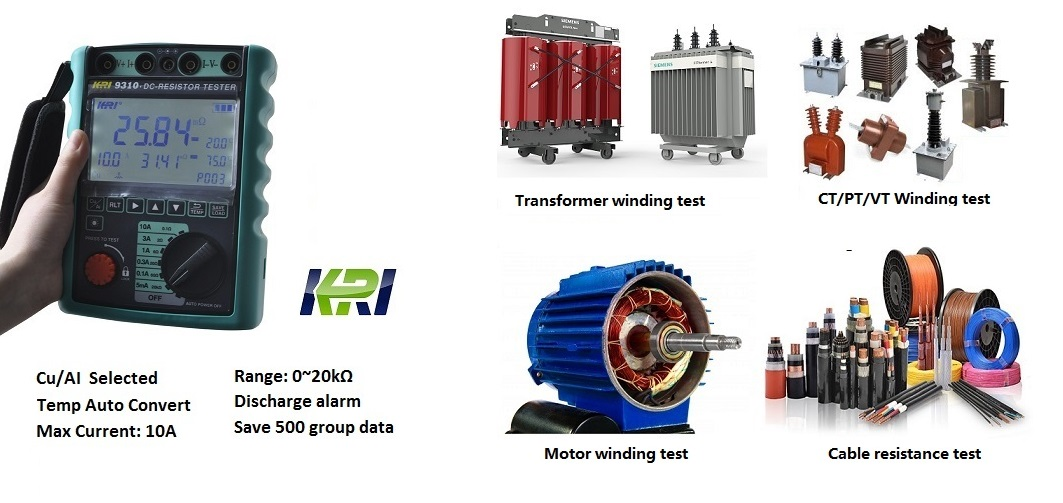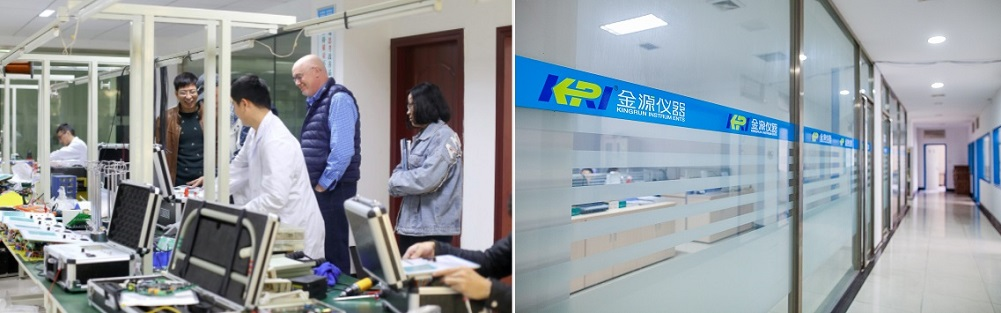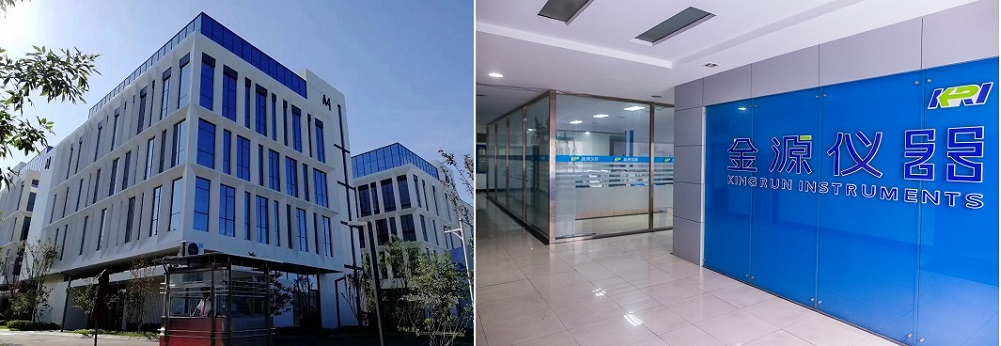Industry News

# Why should the DC Winding resistance of the Transformer or Motor be Converted to 75 °C?

The DC winding resistance of copper and aluminum wires of transformers and motors has a large temperature coefficient. The measurement results of the same winding at different temperatures are quite different. In order to be able to compare at the same temperature, verification, IEC (International Electro technical Commission) The electrical regulations are specified in the direct current group of the motor and transformer windings, the measurement results at different temperatures are converted to the operating temperature (75 ° C).

And not only the DC group of the winding, the insulation resistance of the motor and transformer windings is also converted to the operating temperature (75 ° C).

The simple conversion formula for the factory is:
1, R1 = R2 * (T + Δt1) / (T + Δt2).
2. If it is a copper conductor: T=235; if it is an aluminum conductor: T=225.Δt1 and Δt2 respectively represent the temperature (°C) value of the conductor.
3. If you know that the copper conductor resistance at 20 ° C is R20, then: the resistance of the conductor at 75 ° C:

R75=R20*(235+75)/(235+20)=R20*310/255=1.2157*R20

Kingrun JYR9310 transformer winding resistance tester provides the temperature converting function. Input the tested material( copper /aluminum) and the temperature, the instrument can convert them to the resistance value at the standard temperature (20℃, 75℃ and 120℃)KRI offers a full range of transformer testers to provide professional and accurate testing services for your transformers